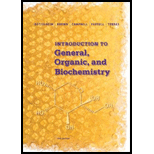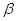# 9-60 The most abundant isotope of uranium, does not undergo fission. Instead, it captures a neutron and emits two particles to make a fissionable isotope of plutonium, which can then be used as fuel in a nuclear reactor. Write an equation for the nuclear reaction and identify the product nucleus.### Introduction to General, Organic a...

11th Edition
Frederick A. Bettelheim + 4 others
Publisher: Cengage Learning
ISBN: 9781285869759### Introduction to General, Organic a...

11th Edition
Frederick A. Bettelheim + 4 others
Publisher: Cengage Learning
ISBN: 9781285869759

#### Solutions

Chapter
Section
Chapter 9, Problem 9.60P
Textbook Problem

## 9-60 The most abundant isotope of uranium,does not undergo fission. Instead, it captures a neutron and emits twoparticles to make a fissionable isotope of plutonium, which can then be used as fuel in a nuclear reactor. Write an equation for the nuclear reaction and identify the product nucleus.

Expert Solution
Interpretation Introduction

Interpretation:

When Uranium-238 captures a neutron it emits two beta particles and forms plutonium-239 which is a fissionable nuclide. The nuclear reaction should be written along with product nuclide.

Concept Introduction:

Total mass number of all nuclides in reactant side must be equal to the total mass number of all nuclides in product side.

Similarly total atomic number of all nuclides in reactant side must be equal to the total atomic number of all nuclides in product side.

### Explanation of Solution

Total atomic number in left hand side is (92+0)=92

Total atomic number in right hand side is (942)=92

Total mass number in

### Want to see the full answer?

Check out a sample textbook solution.See solution

### Want to see this answer and more?

Bartleby provides explanations to thousands of textbook problems written by our experts, many with advanced degrees!

See solution